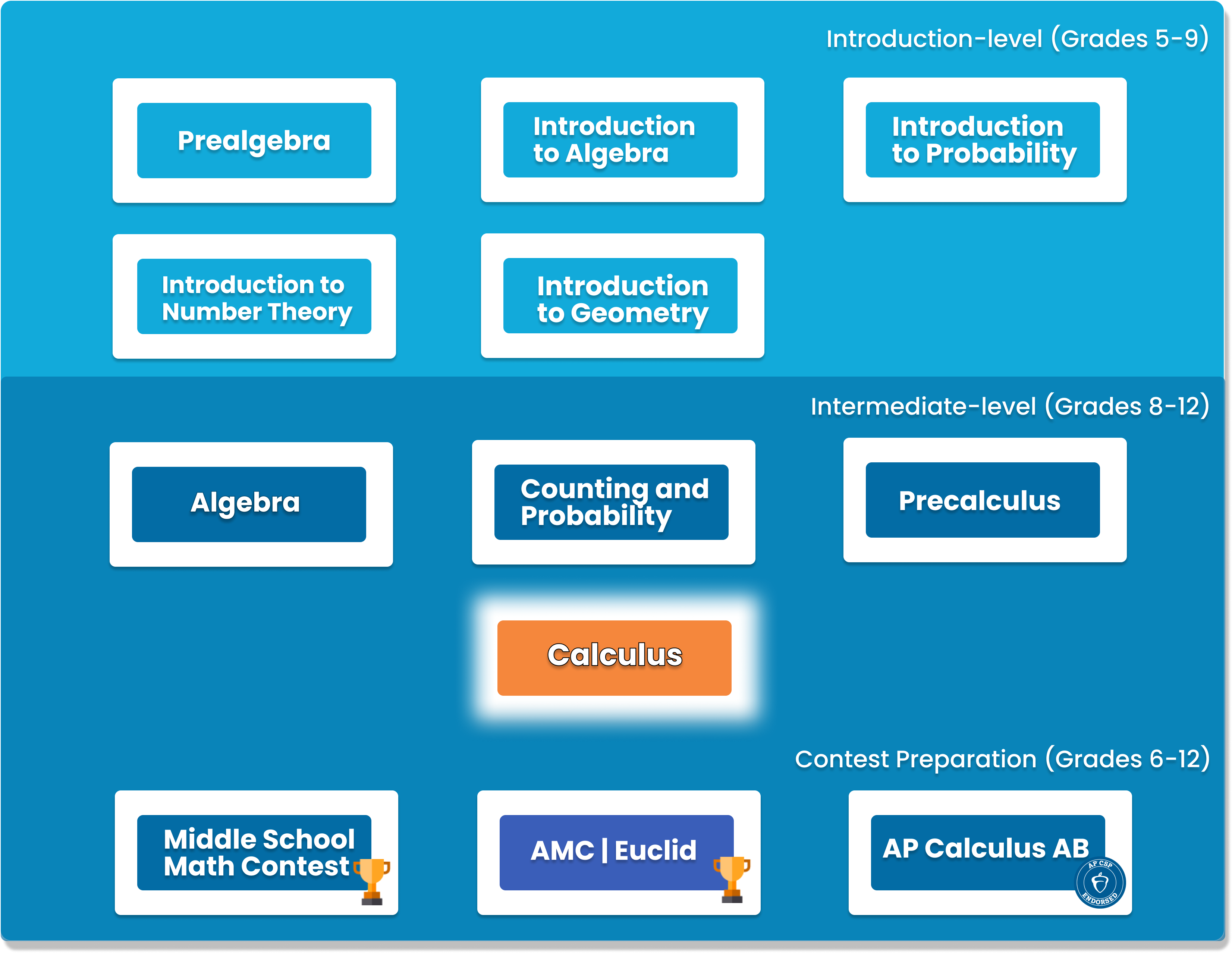# Calculus

This course is a comprehensive study of single-variable calculus. It builds on the topics covered in Pre-Calculus and is the basis for theorem and problem solving that are covered in high school calculus class. It is essential for students looking to further their education with AP Calculus. Specific topics covered include limits, continuity, derivatives, integrals, power series, plane curves, derivative integral series, and differential equations.‍##### Who is this course for
This course is for students who have completed our Pre-Calculus course.
This course typically takes 3-6 months to complete. This depends on the student's experience and how fast they can master the concepts and the knowledge. Our instructors move at the pace of the student, it may take extra time for some students to reinforce what they have learned.
##### Curriculum
###### Chapter 1: Prerequisites for CalculusParametric Equations, Review of Pre-Cal 12, including exponential and logarithms, trigonometric functions
###### Chapter 2: Limits and ContinuityLimits, Continuity, instantaneous Rate of change
###### Chapter 3: DerivativesDefinition, differentiability, rules for differentiation, chain rule, implicit differentiation, derivative of trigonometric, inverse trigonometric, exponential, and logarithms. Applications in kinematics
###### Chapter 4: Applications of derivativesExtreme values of functions, mean value theorem, drawing graph of derivatives, optimization, linearization and newton’s method
###### Chapter 5: Definite IntegralEstimation of integral, definition, antiderivatives, fundamental theorem of calculus
###### Chapter 6: Differential Equations and Mathematical ModelingSlope Fields, integration by substitution and by parts, exponential growth and decay, logistic growth
###### Chapter 7: Applications of definite integralsAreas in the plane, volumes, lengths of curves
###### Chapter 8: Sequences, L’Hopital’s rule, improper integralsSequences, l”hopital’s rule, relative rates of growth, improper integrals
###### Chapter 9: Infinite SeriesPower Series, Taylor Series, Tayler’s theorem, radius of convergence, convergence tests
###### Chapter 10: Parametric, Vector, and Polar FunctionsParametric Functions, Vectors, Polar Functions

### You Might Also Be Interested In Our Elective Courses##### Python Level 1
AGES 10 - 15

Designed for beginners, this level is designed to teach the basic fundamentals and design principles of Python, with the help of Turtle graphics and PyGame. This includes: variables, conditional statements, loop basics, and functions.

These concepts are transferable to any other programming language. Throughout the way, students will create projects in order to apply the concepts they have learned, and to solidify their knowledge.##### Python Level 2
AGES 10 - 15

Level 2 dives deeper into the basics of Python for a more thorough understanding and introduces advanced topics. Students will build on knowledge from Level 1 and work with data structures, advanced loops, algorithms, and object-oriented programming, and create games based on what they learn.

Students will complete the course with a solid understanding of Python fundamentals.##### Java Level 1
AGES 10 - 15

This beginner-friendly course serves as an introduction to the Java programming language. Students will learn the fundamentals of Java along with core computer science concepts. Concepts covered in this course include Java syntax, types and identifiers, operators, if statements, loops, arrays and more.

Students will apply these concepts to create fun games such as Tic-Tac-Toe.